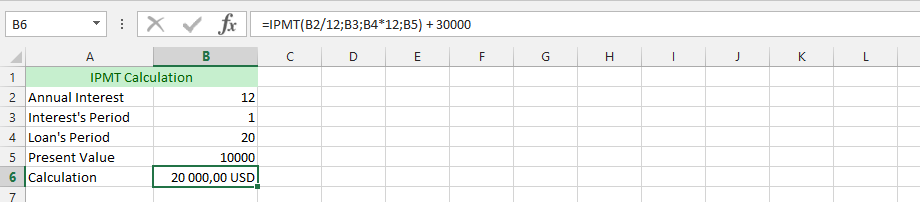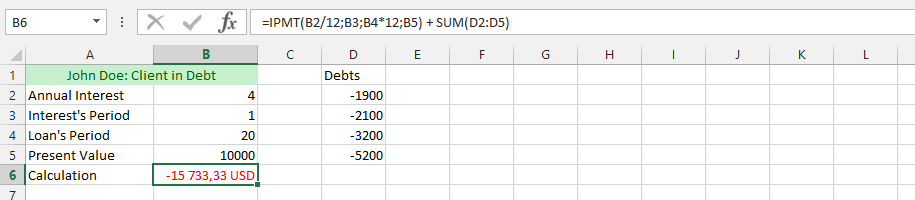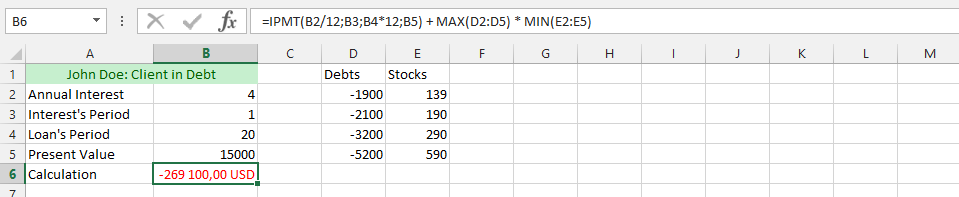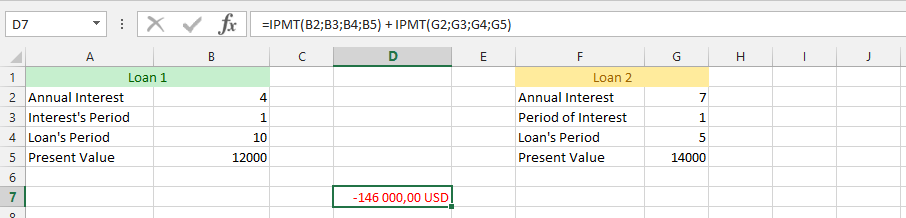### IPMT: Defining And Ways Of Using It

In this lesson you can learn how to use IPMT function in Excel.

This function calculates the amount of interest you have to pay in a given period, assuming that the interest rate and monthly payment in subsequent periods are fixed. Interest with the amount repayable installment loans are periodically calculated using the PMT function

The syntax of this function, the formula is as follows:

=IPMT(rate,per,nper,pv,[fv],[type])

• rate – the interest rate period – the period for which interest is calculated
• per – a period to be determined by a percentage (a range of values is from 1 to nper)
• nper – Total number of payments during the loan repayment or saving
• pv – the current value of the loan taken
• fv – the amount you intend to accumulate in your account after the last payment
• type – 0 is a payment at the beginning of the period and 1 is a payment at the end of the period

Example 1: A Simple IPMT Formula

We are considering lending money to a client. The problem is, we do not know how much the investment would worth in the end. We finds that the IPMT function is the solid tool for finding answers for acknowledging the calculation.Example 2: IPMT with More Details

In our investment bank company, we have a small problem, and we do not know how to best acknowledge it. We are trying to find the comprehensive and more tangible value for IPMT. This allows the possibilities of using the IPMT with a more details.Example 3: Complete IPMT Formula

We have a client with this urge to be more difficult than other clients that we are used to, and would desire putting down some money, while desiring that the value should not worth more than 200,000 in the future. We find this to be extremely difficult, but with the help of IPMT, we can find out how it would work out.Example 4: IPMT Dealing with a Complicated Client

Our client is being quite complicated, with a rare desire. The whole calculation was already set, but the client has come and make a strange demand, which is his ability to add certain amount of money to the down payment. This is why we were going to use the IPMT, and add the amount of money that the client wishes to add to the money. We are using the previous data for finding out, if it would be valuable.Example 5: A Client in Deep Debt

We don’t know if the IPMT would solve the problem, but we belief that it worth a try. The client has multiple debts that he cannot relief. But, he is now considering a new loan that would be combined into the previous loan. He is taking advantage of our new decreased interest rate. For the client, we’d know we need to describe all the debts in the same Excel document for an overview.Example 6: Max and IPMT

The company is considering helping the client. However, we also need to consider or policy that clearly state that the only debt we would accommodate into the new loan policy that the client would be given. The policy states that, we could only accommodate the max of all the respective debts, and the client would have to leave out the remaining debts for something else.Example 7: IPMT, MIN and MAX

We’d have complicated policy that enhance the investment value. This is the perfect place, where we would like to rather operate in a more complicated manner. The business is doing great, and we would know how many stocks their debts would cost us. This complication now lead to us acknowledging the full evaluation of the value. This is why we are now multiplying the max with the min on the IPMT formula.Example 8: IPMT and AVERAGE

The whole business is going quite well. But, this time we’d need to multiply the value of IPMT with the average. This is why we use the IPMT in combination to the interest payment of it, and then multiply it with the average.Example 9: IF and IPMT

This example make use of acknowledgement of using both IF and IPMT to know the result.Example 10: Double IPMT Formula

We need the acknowledgment of interest payment of a client who has two different loan with the company. This is why we are using the IPMT formula, and add them together to know the interest of both, so we would acknowledge the amount of money we would make from both payment interest.Example 11 basic calculation of interest rate

Mr. X took the loan of 20 000 \$ for 2 years. The interest rate is 25% per annum and the loan will be repaid in monthly installments. Calculate the interest in 7th month.

In any cell enter the formula: =IPMT(0.25/12, 7, 12*2, -20000)

• 0.25/12 is interest rate per month
• 7 is a number of installment, because you are looking for seventh installment
• 12*2 is a number of installments- it is 2 years what is 24 months
• -20000 is an amount of credit with minus (thats not your money)

The result is 330,96\$

Example 12 IPMT basic calculation

What is the value of the interest in the first month of the loan amounting to 1 000 000 \$ bearing interest of 8% per annum taken for three years.

In any cell enter the formula: =IPMT(0.08/12, 1, 12*3, -1000000)

• 0.08/12 is interest rate per month
• 1 is a number of installment, because you are looking for first installment
• 12*3 is a number of installments – it is 3 years what is 36 months
• -1000000 is an amount of credit with minus (thats not your money)

The result is 6 666,67\$

Example 13 IPMT calculating for 12th month

What is the value of the interest in the twelfth month of the loan amounting to 2 000\$ bearing interest of 10% per annum taken for five years.

In any cell enter the formula: =IPMT(0.1/12, 12, 12*5, -2000)

• 0.1/12 is interest rate per month
• 12 is a number of installment, because you are looking for twelfth installment
• 12*5 is a number of installments – it is 5 years what is 60 months
• -2000 is an amount of credit with minus (thats not your money)

The result is 14,2\$

Example 14 Bit difficult IPMT to calculate

What is the value of the interest in the eighth week of the loan amounting to 12 000\$ bearing interest of 2% per annum taken for ten years. Payments are due at the beginning of each week.

In any cell enter the formula: <=IPMT(0.02/12, 8, 52*10, -12000, 0, 1)

• 0.02/12 is interest rate per month
• 8 is a number of installment, because you are looking for eighth installment
• 52*10 is a number of installments – it is 10 years what is 52 each year
• -12000 is an amount of credit with minus (thats not your money)
• 0 means that you want pay whole credit
• 1 means that you will pay at the beginning of each period

The result is 19,8\$

Try to calculate amount of the interest when you pay at the end of period. Interest will be higher (but not much here).

## Template

```Further reading: